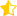Learn from home

The teachersThe angle between a line, r, and a plane, π, is the angle between r and its orthogonal projection onto π, r'.The angle between a line and a plane is equal to the complementary acute angle that forms between the direction vector of the line and the normal vector of the plane.If the line, r, and the plane, π, are perpendicular, the direction vector of the line and the normal vector of the plane have the same direction and therefore its components are proportional:1. Determine the angle between the lineand the plane.2. Determine the angle between the lineand the plane.3. Determine the angle between the line and the plane:Did you like the article?(4 votes, average: 3.25 out of 5)Loading...

Emma

I am passionate about travelling and currently live and work in Paris. I like to spend my time reading, gardening, running, learning languages and exploring new places.

Did you like
this resource?

Bravo!